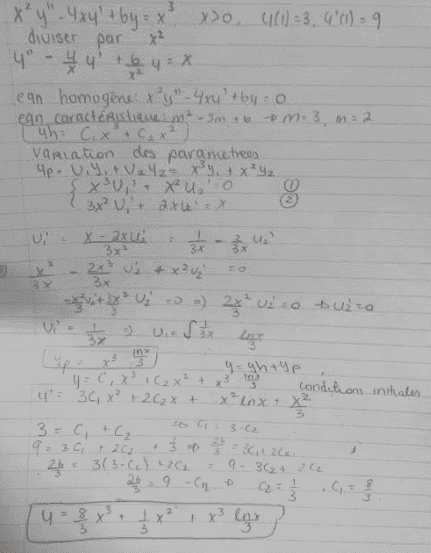# Second order non homogeneous ODE, IVP

• Cocoleia

## Homework Statement

I need to solve:
x^2y''-4xy'+6y=x^3, x>0, y(1)=3, y'(1)=9

## The Attempt at a SolutionI know that the answer is: y=x^2+2x^3+x^3lnx
Where did I go wrong. I was wondering if it's even logical to solve it as an Euler Cauchy and then use variation of parameters...

Last edited: